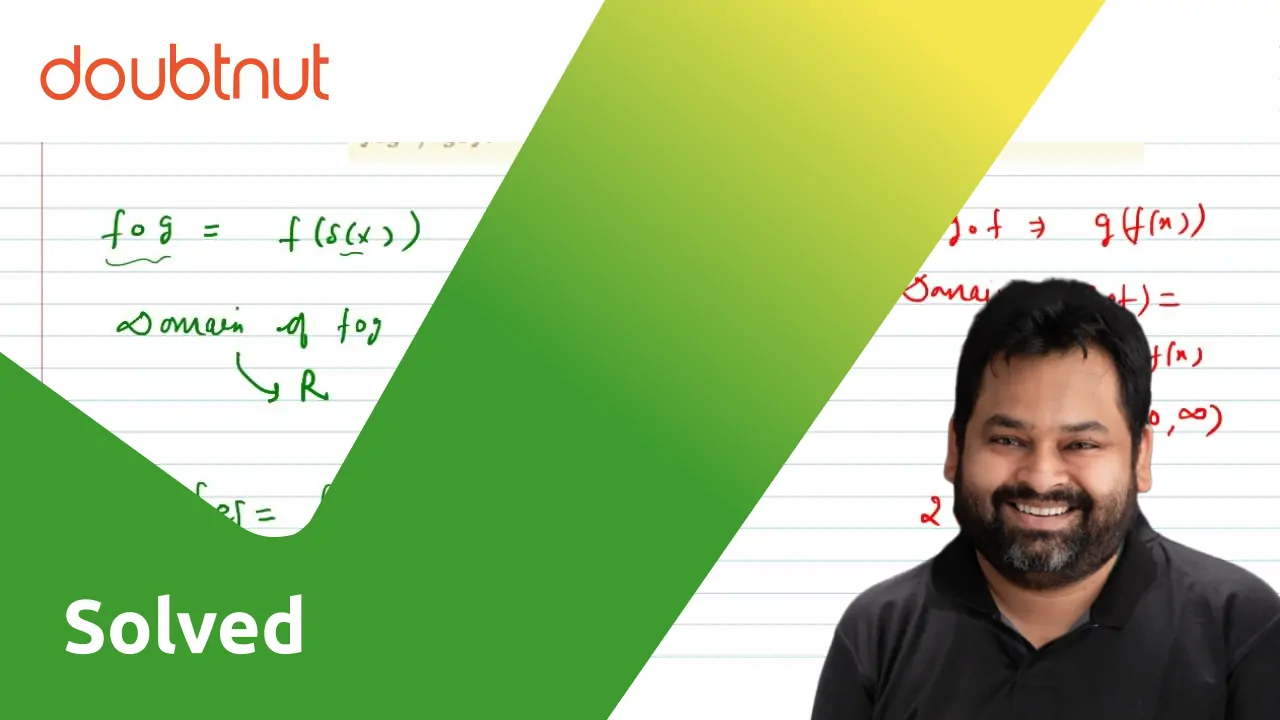Doubtnut App - Get 50 Lakh+ video solutions better than GoogleInstall Now

# Let R be the set of real numbes. If f:R→R;f(x)=x2 and g:R→R;g(x)=2x+1 . Then, find fogandgof . Also, show that fog≠gof.

Text Solution
Verified by Experts

## f:R→g:R→R =x2,g(x)=2x+11[g(x)]=[g(x)]2flog=(2x+1)2 g(x)=2x+1 g[f(x)]=2f(x)+1 gof=2x2+1 fog≠gof.